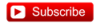# YouTubeT4-36: Fixed Income: Simple bond illustrating all three durations (effective, mod, Mac)

#### Nicole Seaman

##### Director of FRM Operations
Staff member
Subscriber
Macaulay duration is the bond's weighted average maturity (where the weights are each cash flow's present value as a percent of the bond's price; in this example, the bond's Macaulay duration is 2.8543 years. Modified duration is the true (best) measure of interest rate risk; in this example, the modified duration is 2.7847 years. We use modified duration to estimate the price impact given a parallel shock to the yield curve: given Δy of X%, we estimate an D*X% = Δ%P change in the bond's price. In this example, a 1.0% increase in yield anticipates a 1.0% * 2.7847 = -2.7847% price drop. It's important to keep in mind that duration represents only the linear approximation (i.e., convexity is omitted) so as the yield shock increases, its estimate becomes less accurate. Effective duration is used to approximate modified duration when it is not easy to access analytically. As an estimate of modified duration, effective duration has the same use/purpose as modified duration.